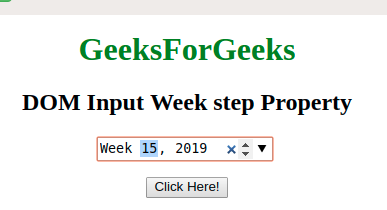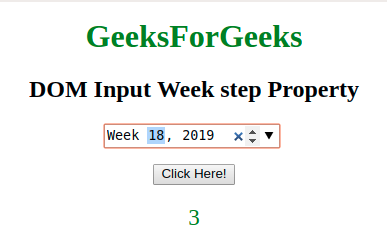# HTML | DOM Input Week step Property

• Last Updated : 19 Jan, 2022

The DOM Input Week step Property is used to set or return the value of the step attribute of a week field. The step attribute in HTML is used to set the discrete step size of the element. The default stepping value for week inputs is 1.
The step attribute could be used with min and max attribute for creating a legal value.

Syntax:

• It return the step property.
`weekObject.step`
• It is use to Set the step property.
`weekObject.step = number`

Property Values: It contains a value i.e number Which specify the legal weeks interval for the week field. It has a default value which is 1.

Return Value: It returns a numeric value which represents the legal week’s interval for the week field.

Example-1: This example illustrates how to return the Property.

 ```<``html``>`` ` `<``body` `style``=``"text-align:center;"``>`` ` `    ``<``h1` `style``=``"color:green;"``> ``            ``GeeksForGeeks ``        ```` ` `    ``<``h2``>``      ``DOM Input Week step Property``  ```` ` `    ``<``input` `type``=``"week"` `           ``id``=``"week_id"` `           ``step``=``"3"``           ``placeholder``=``"Every 3rd week"``>``    ``<``br``>``    ``<``br``>``    ``<``button` `onclick``=``"myFunction()"``>``        ``Click Here!``    ```` ` `    ``<``p` `id``=``"gfg"` `       ``style="font-size:23px;``              ``color:green;">``  ```` ` `    ``<``script``>``        ``function myFunction() {`` ` `            ``// Accessing input value ``            ``var x =``                ``document.getElementById(``                  ``"week_id").step;``           ` `            ``document.getElementById(``              ``"gfg").innerHTML = x;``        ``}``    ```` ` ``` ` ``

Output:(Every 3rd week from the 1st week can be selected only)
Before clicking on button:After clicking on button:Example-2 : This example illustrates how to set the property.

 ```<``html``>`` ` `<``body` `style``=``"text-align:center;"``>`` ` `    ``<``h1` `style``=``"color:green;"``> ``            ``GeeksForGeeks ``        ```` ` `    ``<``h2``>``      ``DOM Input Week step Property``  ```` ` `    ``<``input` `type``=``"week"` `           ``id``=``"week_id"``           ``step``=``"5"``           ``placeholder``=``"multiples of 5"``>``    ``<``br``>``    ``<``br``>``    ``<``button` `onclick``=``"myFunction()"``>``        ``Click Here!``    ```` ` `    ``<``p` `id``=``"gfg"` `       ``style="font-size:23px;``              ``color:green;">``    ```` ` `    ``<``script``>``        ``function myFunction() {`` ` `            ``// set step value ``            ``var x =``                ``document.getElementById(``                    ``"week_id").step = "3";`` ` `            ``document.getElementById(``                    ``"gfg").innerHTML =``                ``"The value of the step " +``                ``"attribute was changed to " + x;``        ``}``    ```` ` ``` ` ``

Output:
Before clicking on button:After clicking on button:Note : In Firefox, the input type=”week” element does not show any date field or calendar.

Supported Browsers: The browser supported by DOM Input week step Property are listed below: